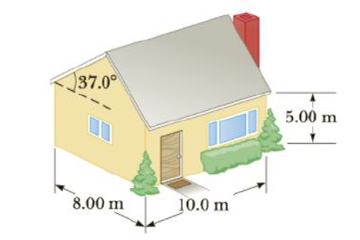Chapter 11, Problem 46P

Chapter
Section
Textbook Problem

The average thermal conductivity of the walls (including windows) and roof of a house in Figure P11.46 is 4.8 · 10−4 kW/m · °C, and their average thickness is 21.0 cm. The house is heated with natural gas, with a heat of combustion (energy released per cubic meter of gas burned) of 9 300 kcal/m3. How many cubic meters of gas must be burned each day to maintain an inside temperature of 25.0°C if the outside temperature is 0.0°C? Disregard surface air layers, radiation, and energy loss by heat through the ground.Figure P11.46

To determine
The amount of gas must be burned each day to maintain a temperature.

Explanation

Section1:

To determine: The total surface area of the house.

Answer: The total surface area of the house is 304m2 .

Explanation:

Given info: The length of the side wall is 10.0 m, length of the end wall is 8.00 m and the height of the side wall is 5.00 m, the roof inclination angle is 37.0° .

Formula to calculate the area of the sidewalls is,

Aside=2(lsh) (1)

• Aside is the area of the sidewalls,
• ls is the length of the sidewall,
• h is the height of the sidewall,

Formula to calculate the area of the end walls is,

Aendwalls=2(leh) (2)

• Aendwalls is the area of the end walls,
• le is the length of the end wall,
• h is the height of the sidewall,

Formula to calculate the area of the gables is,

Agables=2[12(lehg)] (3)

• Agables is the area of the gables,
• le is the length of the end wall,
• hg is the altitude of the gables,

Formula to calculate the area of the roof is,

Aroof=2(lswr) (4)

• Aroof is the area of the roof,
• wr is the width of the roof,

The total area of the house is,

A=Aside+Aendwalls+Agables+Aroof (5)

Use (I),(II),(III),(IV) in (V) to rewrite A.

A=2(lsh)+2(leh)+2[12(lehg)]+2(lswr) (6)

From the figure

hg=(le2)tan37.0° (7)

wr=(le/2)cos37.0° (8)

Use (7) and (8) in (4) to rewrite A.

A=2(lsh)+2(leh)+2[12(le(le2)tan37.0°)]+2(ls(le/2)cos37.0°)

Substitute 10.0 m for ls , 8.00 m for le , and 5.00 m for h to find A.

A={2[(10.0m)(5.00m)]+2[(8.00m)(5.00m)]+2[12((8.00m)((8.00m)2)tan37.0°)]+2((10.0m)((8

Still sussing out bartleby?

Check out a sample textbook solution.

See a sample solution

The Solution to Your Study Problems

Bartleby provides explanations to thousands of textbook problems written by our experts, many with advanced degrees!

Get Started

Why do we use different map projections?

Fundamentals of Physical Geography

Calculate your protein RDA.

Understanding Nutrition (MindTap Course List)

List the differences between mitosis and meiosis in the following chart:

Human Heredity: Principles and Issues (MindTap Course List)